# Module 1: INITIAL SETUP AND BASIC OPERATION

## Learning Objectives

After reading this lesson, you should be able to:

1. open and name a new m-file,

2. use the m-file to be able to solve mathematical problems,

3. change and manipulate data in the m-file,

4. use comments in an m-file.

## What is an m-file?

Just like how you may write a letter in a word processor program, one writes a MATLAB program in an m-file. This type of file is unique to MATLAB, but not much different than a text file which one could write or edit in text editors such as Notepad.

The m-file brings a whole new level of organization and ease of modifications to MATLAB that the Command Window cannot offer. The m-file and the Command Window are independent of each other but they communicate like best friends. The m-file is where all commands will be input, saved and then “run” through MATLAB, which evaluates the program and outputs the results in the Command Window. Changing any input, command, variable name or number in the m-file is easily done and does not require much time or effort. This is unlike the Command Window where if you have performed, for example, fifty statements, changing the first statement can be very difficult and time-consuming. The m-file can also be saved by the user to any directory, and reused or changed later. You can see examples of how this saved file (m-file) looks in the Windows File Explorer in Figure 1.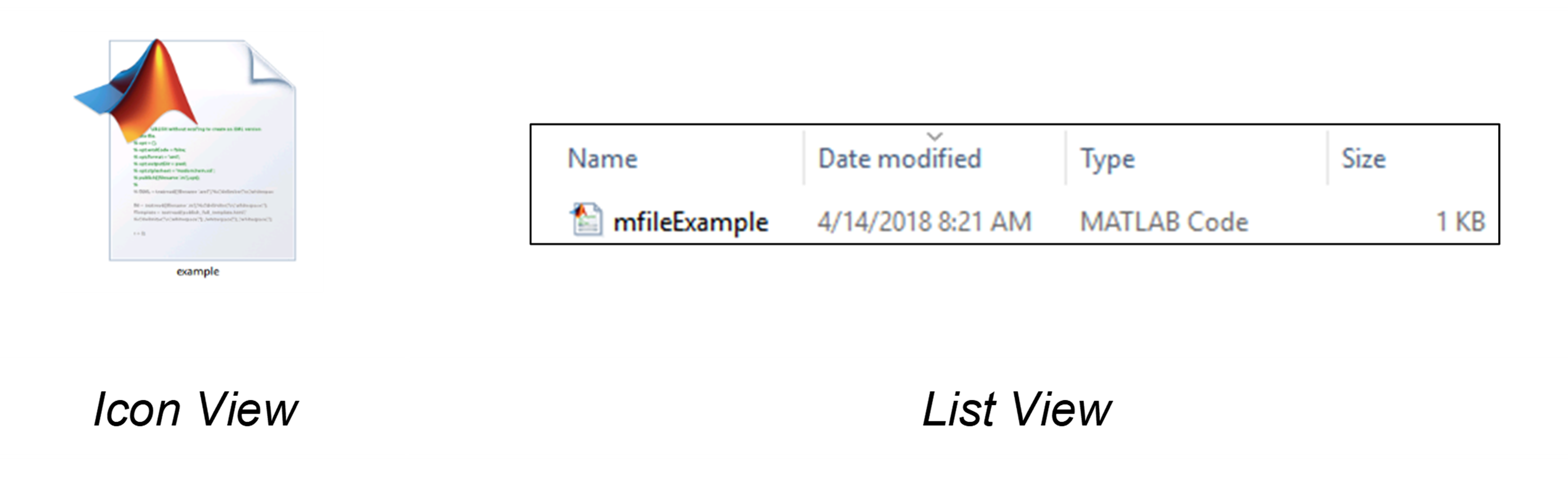Figure 1: This is what the m-file will look like in a file explorer (Windows in this case).

**Where can I create a new m-file?

First, open MATLAB. Now, to open a new m-file, click the “Home” tab in the top left corner of the MATLAB window (see Figure 2). Finally, click the “New Script” button. Note that this is the same procedure we went through in the Hello World lesson. A new window is now open in the MATLAB software; this is the m-file editor. You can change how these windows (Editor, Command Window, etc.) look in MATLAB, which we covered in Lesson 1.4.Figure 2: Click “New Script” on the far left under the “Home” tab to create a new m-file.

## How do I save my m-file?

One of the most important things you can do in MATLAB is to save your m-file correctly. You can save your work by clicking the “Editor” tab, then selecting “Save”. You should make sure to save it under a name that makes sense to you. Do not keep the m-file name as the default “untitled1.m” as this can become very confusing later when you need to find the correct program. Follow the rules below for saving all m-files.

1. Do not start the m-file name with a number.

2. Do not place any spaces in the name of your m-file.

3. Do not use special characters in the name of your m-file such as *, /, +, \$, %, &, #, @, etc. Underscores are permitted in m-file names.

4. Do not use any predefined commands to name the m-file.

5. Do name the m-file something that makes sense to you.

## How do I input variables and expressions into the m-file?

MATLAB always reads the information in the m-file from top to bottom and from left to right (like a book). Also, each line of the m-file has the variable side and an expression side separated by an equal to sign. This is called an assignment and should not be confused with what we call an equation. If, for example, the lengths of two sides of a rectangle, a and b, are 2 inches and 6 inches, respectively, just type:

a = 2

b = 6

The data is now part of the m-file. Then, if we want to find the area of that rectangle, all we would need to do is type:

area = a*b

What we just did was type three lines of code, each line containing an assignment and altogether making a program. For MATLAB to run the program, and for the programmer to receive an output, the m-file must now be “run”.

## How do I run the m-file?

Running an m-file can be done by clicking on the “Editor” tab in MATLAB navigation ribbon, followed by clicking on “Run” (Figure 3). MATLAB will now execute the m-file and perform whatever code is specified, and then display the outputs in the Command Window. This is where the two independent windows communicate with each other; the outputs of the m-file are sent to the Command Window to be displayed. Figure 4 illustrates this for the example of finding the area of a rectangle.Figure 3: Run the program by click the highlighted button under the “Editor” ribbon tab (top).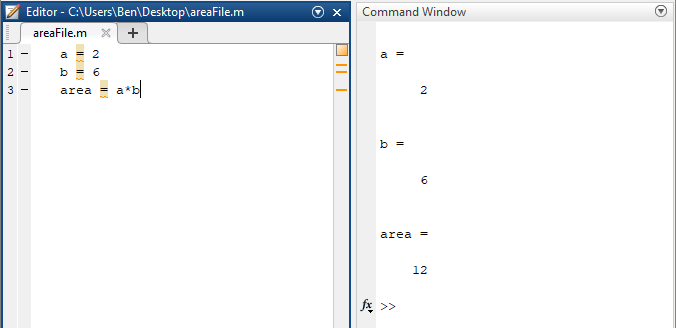Figure 4: Using the m-file to solve for the area of a rectangle and displaying the results in the Command Window.

Go ahead and make a few changes to the m-file and run it again. Notice that the original information is still displayed in the Command Window. If we are going to run this program many times with different inputs, we would want to overwrite the old information and only display the up-to-date information in the Command Window. We can do this by using the clc and clear commands.

## What are the clc and clear commands?

The clc command stands for clear Command Window. This command is used and placed in the m-file (it may be used in the Command Window too), and all the information preceding it gets cleared in the Command Window once the m-file is run. The clear command clears the assignment of all variables from a previous session (more specifically from the MATLAB “workspace”). Together, both of these commands provide for a smooth operating m-file, and it is considered good programming practice to make these commands the first two lines of your m-file. Example 1 shows the clc and clear commands being used in an m-file, and you will see this usage through the rest of the textbook.

## How can I place comments in my m-file?

When you are writing a program, especially one that contains a large amount of information, placing notes (called comments) to yourself or anyone else that reads the code is beneficial. The MATLAB command to create a comment is the percent sign (%) followed by whatever text is required for the comment. Placing comments is essential and should be used to provide descriptions for the variables, expressions, etc. in the m-file. Example 1 shows the use of comments in an m-file. Note that comments do not affect the code and are not displayed in the Command Window (comments are non-executable code). To display a note in the Command Window, another command is required (see Lesson 2.4).

### Example 1

Put a “comment” in MATLAB.

Solution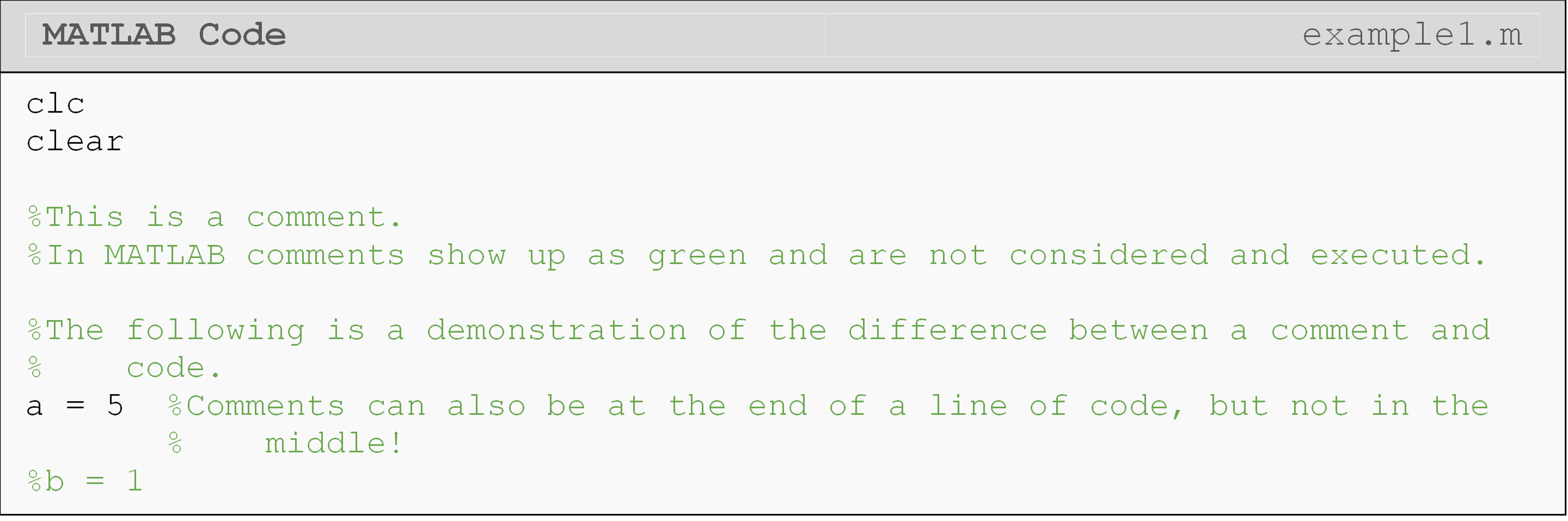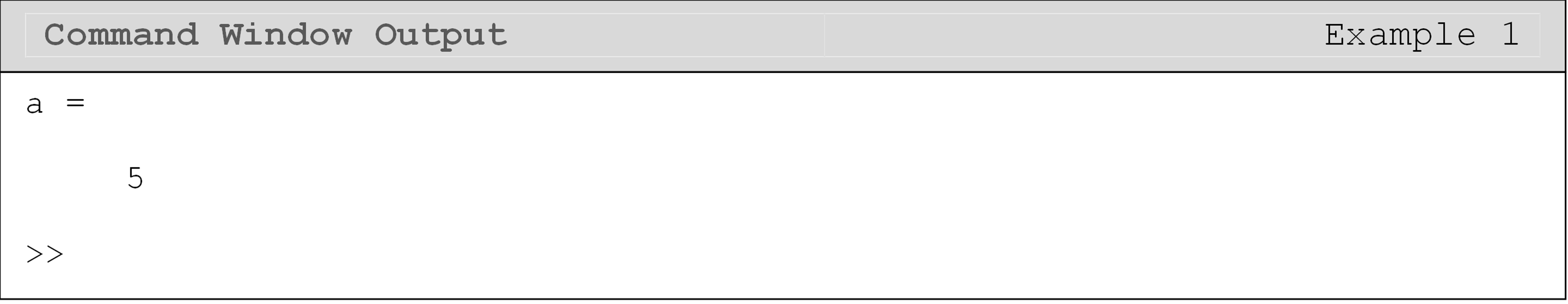## Can I separate my code into parts within the m-file?

MATLAB offers a special kind of comment called a section. You can think of sections like a paragraph in an essay. It is useful to visually separate paragraphs (sections), but they are part of the whole essay (program). To create a section in an m-file, simply type ‘%%’. Notice the mandatory space after the two comment characters. Sections allow you to do several useful things within your m-file, including:

1. using the “Run Section” feature, which only runs the current section > (wherever you last clicked). You can see this option right next to > the “Run” button in the Editor tab (Figure 5). Note that clicking > “Run” still runs the whole m-file as it normally would.

2. creating a more organized “published” m-file. You can see more > details about the published files and how to make them in Lesson > 1.7.

3. organizing your code within your m-file. Sections provide visual > divisions within the m-file as well. As seen in Figure 5, a > horizontal line gets placed at the top of a section, and the > active section is highlighted with a yellowish background.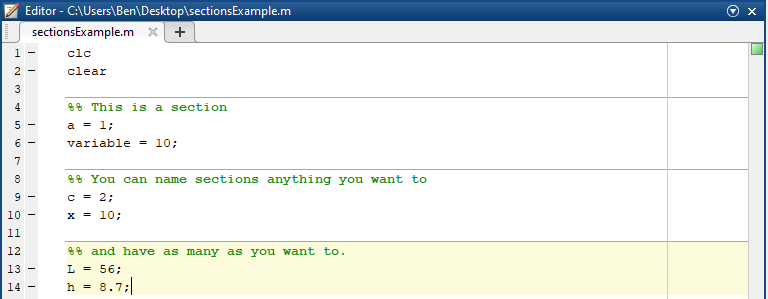Figure 5: Using sections in an m-file.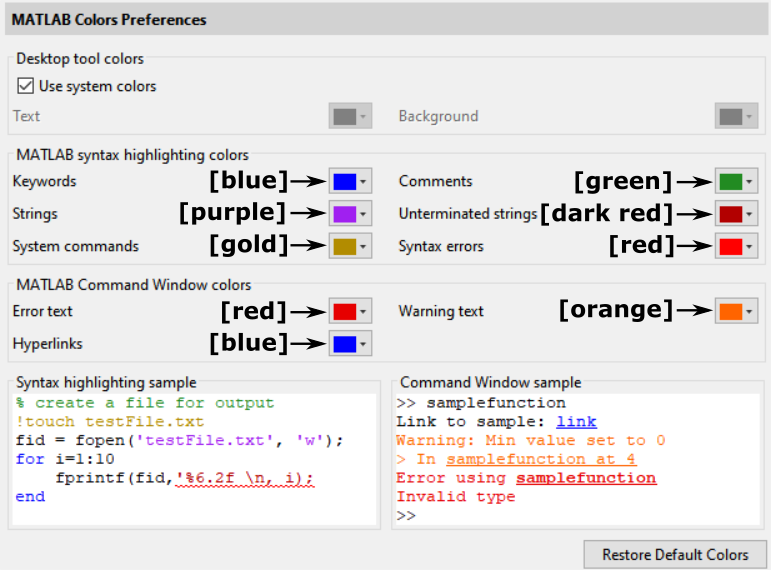Figure 6: Default color highlighting preferences in MATLAB.

## What does the color highlighting in the m-file mean?

By now, you have noticed that MATLAB highlights some text in different colors. In Figure 6, you can see the default color preferences in MATLAB which describe how MATLAB color codes different text. You can see comments are selected as green.

It is OK if you do not know what all of the terms mean (like “strings”) in Figure 6 as we will cover these in later lessons and all examples are color-coded appropriately. This is just to introduce you to the idea of color-coding in MATLAB. You can find more information on the color settings page of MATLAB.

## Lesson Summary of New Syntax and Programming Tools

 Task Syntax Example Usage Clear the command window clc clc Clear one or all variables clear clear Add an m-file comment % % Any text can go here

## Multiple Choice Quiz

(1). The clc command is used to

(a)  clear the command window.

(b)  erase everything in the m-file.

(c)  delete all saved (workspace) variables.

(d)  save the existing m-file.

(2). The command to place a comment into the m-file is

(a)  %

(b)  !

(c)  ;

(d)  &

(3). Which m-file is named correctly

(a)  1assignment.m

(b)  assignment 1.m

(c)  assignment1.m

(d)  assignment/1.m

(4). The clear all command is used to

(a)  clear the command window.

(b)  erase everything in the m-file.

(c)  delete all saved (workspace) variables.

(d)  save the existing m-file.

(5). Clicking “Run” in MATLAB in an m-file with five sections runs

(a)  the whole m-file.

(b)  only the first section of the m-file.

(c)  nothing because “Run” does not work with an m-file containing sections.

(d)  only the last section of the m-file.

## Problem Set

(1). The weight of an object can be found using

$w = m \times g$

where,

$$w = \text{weight }(N)$$

$$m = \text{mass of the object (kg)}$$

$$g = \text{gravity }\left( \text{m/sec}^{2} \right)$$

Write an m-file to find the weight of a 30 kg Martian on earth (g=9.8 m/sec2) and on Mars (g=3.7 m/sec2). Make sure to suppress intermediate outputs and write comments in the m-file.

(2). You are asked to find the volume of a cylindrical storage tank. You know that the interior diameter is 3 feet and the length is 5 feet. Write a MATLAB m-file that finds the volume of this tank. Your boss needs the Command Window to look nice, so be sure to suppress intermediate outputs and only show the final answer in the Command Window.

(3). Congratulations! You’ve just been hired at The Pressure is on Us, Inc. Your first assignment is to write a MATLAB program that finds the pressure inside a cylindrical storage tank. You know that the tank has a 2.15 m interior diameter and is 4.55 m in length. You also know that the tank will hold 215 kg of an ideal gas at 400 K. The pressure in the tank can be found using:

$p = \frac{mRT}{V}$

where,

p is pressure (Pa)

m is mass (kg)

V is volume (m$$^3$$)

T is temperature (K)

R is the ideal gas constant, $$287.05\ \displaystyle\frac{\text{N}\cdot \text{m}}{\text{kg}\cdot \text{K}}$$

Follow the expected rules of display of Command Window and comments in m-file.

(4). A 12-volt battery and a switch are placed in parallel with the primary windings of a transformer. The secondary windings are placed in parallel with a resistor (See Figure A). Find the current traveling through the resistor after the switch is closed.

You may use

$$\displaystyle\frac{n_s}{n_p} = \frac{V_s}{V_p}$$, and $$\displaystyle I = \frac{V}{R}$$

where,

$$n_s=\text{number of secondary windings}$$

$$n_p=\text{number of primary windings}$$

$$V_p=\text{primary voltage (V)}$$

$$V_s=\text{secondary voltage (V)}$$

$$I=\text{current (A)}$$

$$R=\text{resistance}\ (\Omega)$$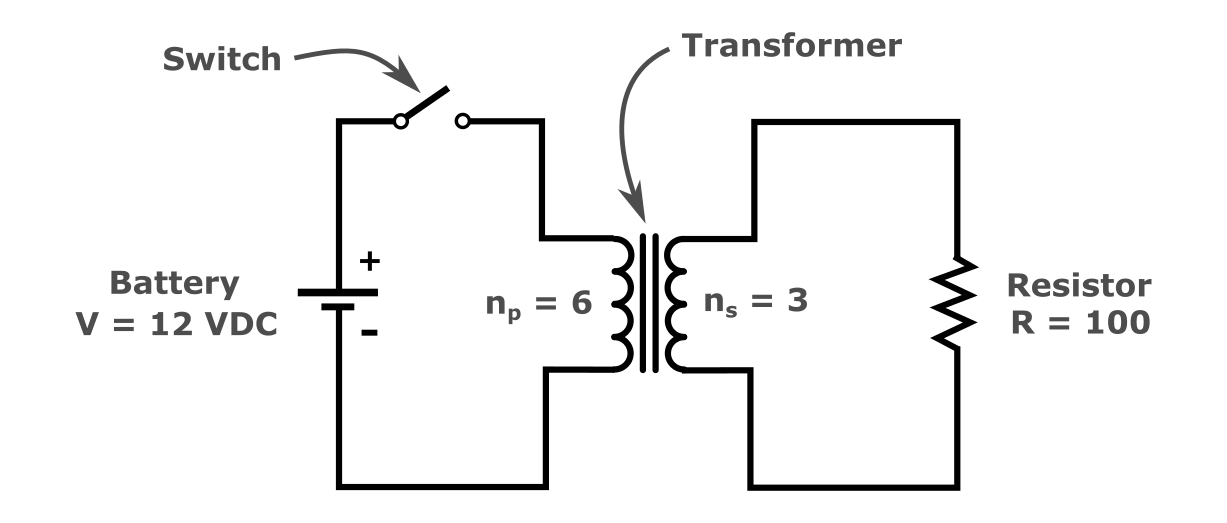Figure A: Electrical Schematic for Exercise 4.

(5). The applied normal stress on an object is, in general, defined as the force acting on the object divided by the area the force is acting on. Assuming that a force, $$F = \text{252.2 lbs}$$ is acting in the direction as shown (see Figure B), find the normal stress in the body that has the top cross-sectional area of $$a = 2\text{ i}\text{n}^{2}$$.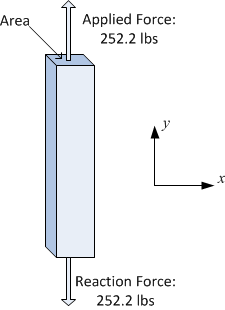figure22e_stress

Figure B: Object with a force acting on its top face.

(6). When a normal axial load is applied to a thin plate with a hole, the nominal stress at the hole is amplified by a factor called the stress concentration factor, $$k_t$$. The maximum stress $$\sigma_{\max}$$is given by

$\displaystyle\sigma_{max}=k_t\sigma_{nom}$

where,

$$\sigma_{max} =$$ the maximum stress in the body,

$$k_t =$$ the stress concentration factor

$$\sigma_{nom} =$$ the nominal stress.

For a thin plate with a center hole, the stress concentration factor $$k_t$$ is

$k_{t} \approx 3.0039 - 3.753\frac{D}{W} + 7.9735\left( \frac{D}{W} \right)^{2} - 9.2659\left( \frac{D}{W} \right)^{3} + 1.8145\left( \frac{D}{W} \right)^{4} + 2.9684\left( \frac{D}{W} \right)^{5}$

where,

$$W$$ is the width of the plate

$$D$$ is the diameter of the hole

If a force, $$F$$ of 1230 lbs is applied to a thin plate (see Figure C), find the peak stress in the plate. The width of the plate is 30 inches, the diameter of the hole is 1.25 inches, and the plate thickness is 1.25 inches.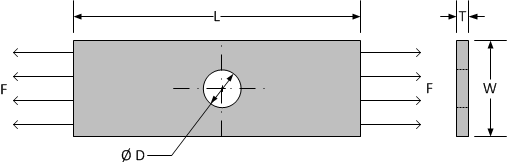figure23e_wholestress

Figure C: Thin plate shown with applied load, F for Exercise 6.

Hint: To find the nominal stress you may use,

$$A_{nom}=(W-D)\times T$$

$$\sigma_{nom}=\displaystyle\frac{F}{A_{nom}}$$